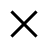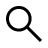Buscar

# How to calculate the space occupied by tables and indexes in SQL ServerWho I am
Martí Micolau
@martimicolauAuthor and references

Sometimes there is a need to do a little bit of cleaning, or at least understand why our database is so large.

Here is a script that allows you to locate table by table, index by index the number of records and occupied disk space.

## How to calculate the space occupied by tables and indexes in SQL Serverdeclare @SourceDB varchar(128)
declare @sql varchar (128)
create table #tables(name varchar(128))
select @sql = ‘insert #tables select TABLE_NAME from INFORMATION_SCHEMA.TABLES where TABLE_TYPE = ”BASE TABLE”’
exec (@sql)

create table #SpaceUsed (name varchar(128), rows varchar(11), reserved varchar(18),
data varchar(18), index_size varchar(18), unused varchar(18))
declare @name varchar(128)
select @name = ”
while exists (select * from #tables where name > @name)
begin
select @name = min(name) from #tables where name > @name
select @sql = ‘exec ..sp_executesql N”insert #SpaceUsed exec sp_spaceused ‘ + @name + ””
exec (@sql)
end
select Name NomeTabella,
cast([rows] as decimal) righe,
cast(left(reserved,len(reserved)-3) as decimal(18,2))/1024 riservato,
cast(left(data ,len(data)-3) as decimal(18,2))/1024 Dati,
cast(left(index_size ,len(index_size)-3) as decimal(18,2))/1024 Indici,
cast(left(unused ,len(unused)-3) as decimal(18,2))/1024 NonUsato
from #SpaceUsed
order by riservato desc
drop table #tables
drop table #SpaceUsed

### Related Items

##### add a comment of How to calculate the space occupied by tables and indexes in SQL Server
Comment sent successfully! We will review it in the next few hours.